# CBSE Class 12 Chemistry Exam 2018: Question Paper Analysis

The Central Board of Secondary Education (CBSE) successfully conducted the Class 12 Chemistry Exam on 13th March 2018 from 10:30 a.m. to 1:30 p.m. Around 5 lakh students appeared for the exam this year. Chemistry is a very important subject for Class 12 science students. Students were given 15 minutes to go through the exam paper before writing the answers, so the chemistry paper was distributed at 10:15 a.m.

### Question Pattern

The CBSE Class 12 Chemistry exam consisted of 5 sections viz. A, B, C, D and E which consisted a total of 70 marks with a time duration of 3 hours. The detailed CBSE Class 12 Chemistry question pattern is given in the table mentioned below.

 Section No. Of Questions Marks of Each Question Total Marks A 5 1 5 B 5 2 10 C 12 3 36 D 1 4 4 E 3 5 15 Total – 26 70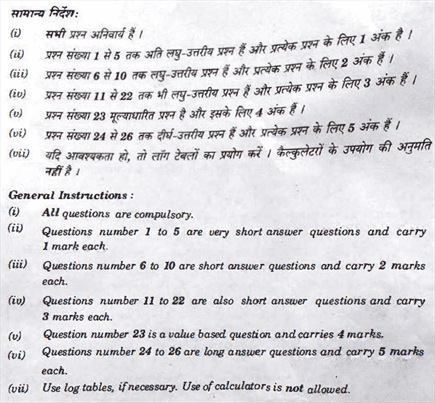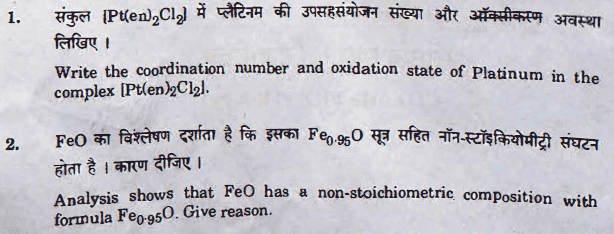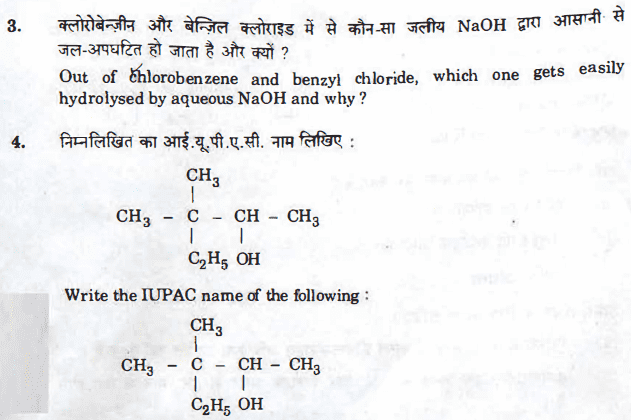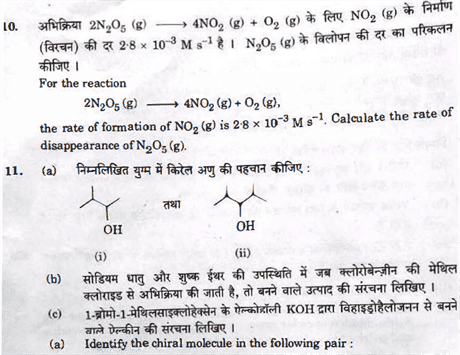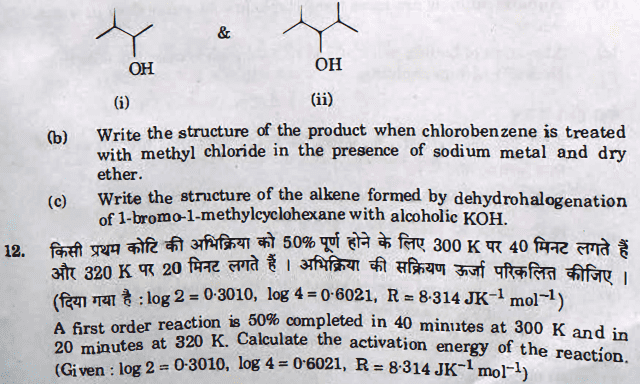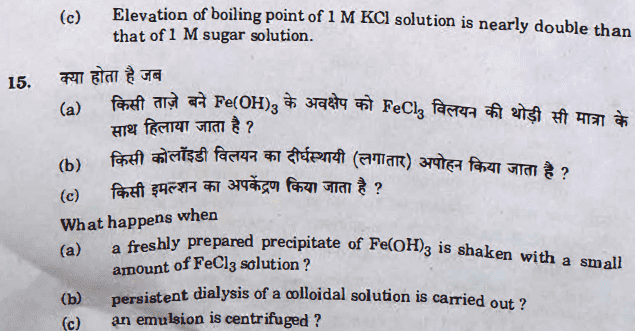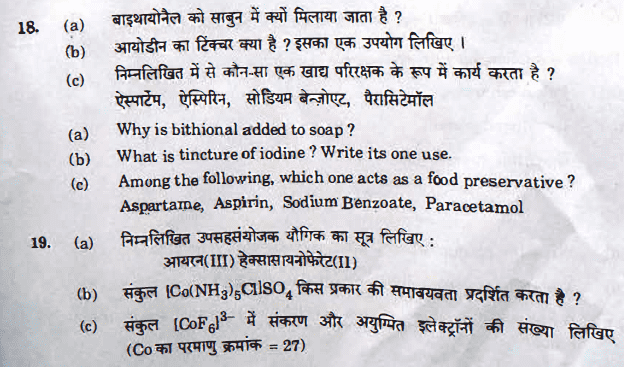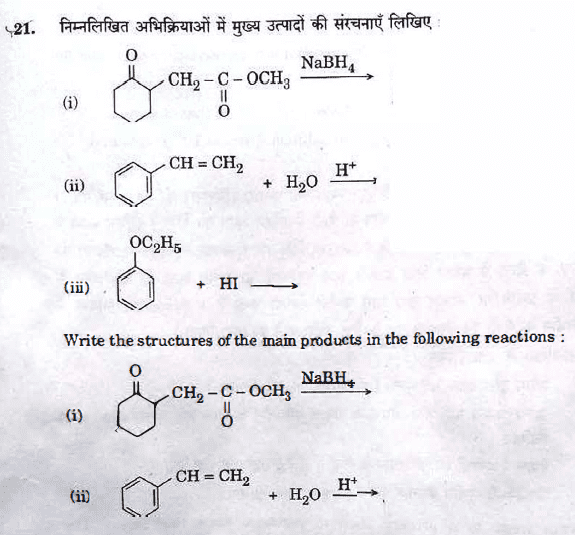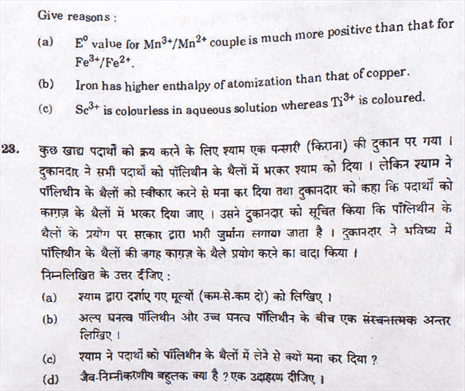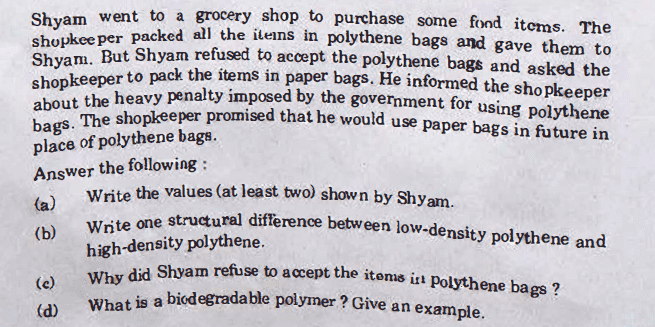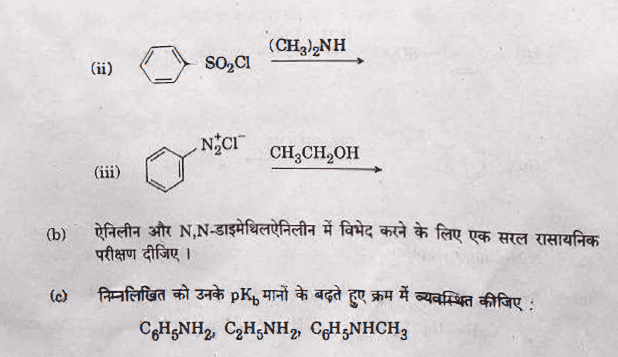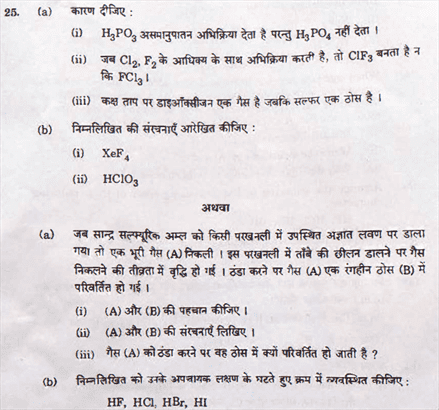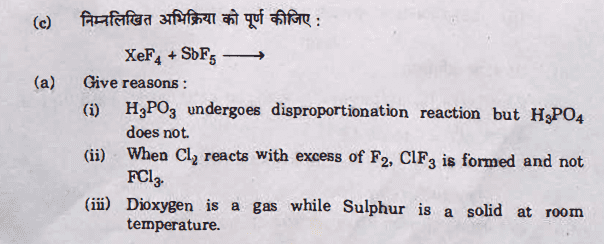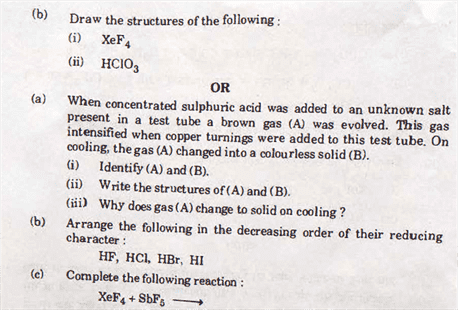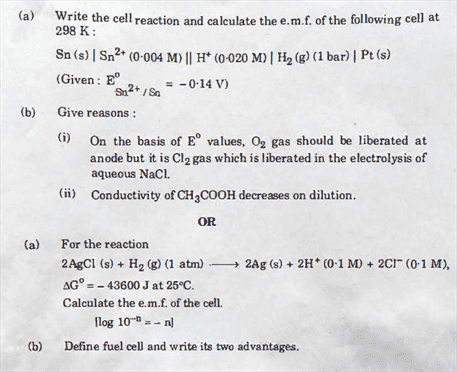### Chapter-Wise Marks Weightage

According to most students, the organic chemistry questions were easier than the previous year. Not a single question was asked from d and f block in this years chemistry question paper. Surface Chemistry has reasoning type questions and not definition type question like last year. All the questions in the exam were asked from the CBSE Class 12 Chemistry syllabus.

The chapter wise marks weightage along with the comparison with last years paper is given in the table below.

 Topic Name Marks Weightage (2017) Marks Weightage (2018) The Solid State 2 1+3=4 Electrochemistry 2+3=5 3+5=8 Solutions 5 2+3=5 Surface Chemistry 1+3=4 1+3=4 Chemical Kinetics 1+3=4 2+3=5 The p-Block Elements 1+2+3 =6 2+2 + 5 = 9 General Principles and Processes of Isolation of Elements 3 3 Coordination Compounds 2+3 =5 1+3 = 4 The d- and f-Block Elements 5 – Alcohols, Phenols and Ethers 1+2 =3 1+ 3 = 4 Haloalkanes and Haloarenes 1+3 =4 1+3 = 4 Amines 3 5 Aldehydes, Ketones and Carboxylic Acids 3+5=8 2+3=5 Polymers 3 4 Biomolecules 4 3 Chemistry in Everyday Life 3 3